# 348. A-Level colouring (Updated)

Those of you who follow this blog will know I have a thing for explaining with colours. This isn’t just a gimmick for younger students, it also works for 16-18 year olds.

In the picture below we were looking at proving a statement involving reciprocal trigonometric functions and fractions. A common source of misconception with this kind of question is that students split the question into working with the numerator and denominator separately, then make mistakes when they put them back together. They can’t see the big picture.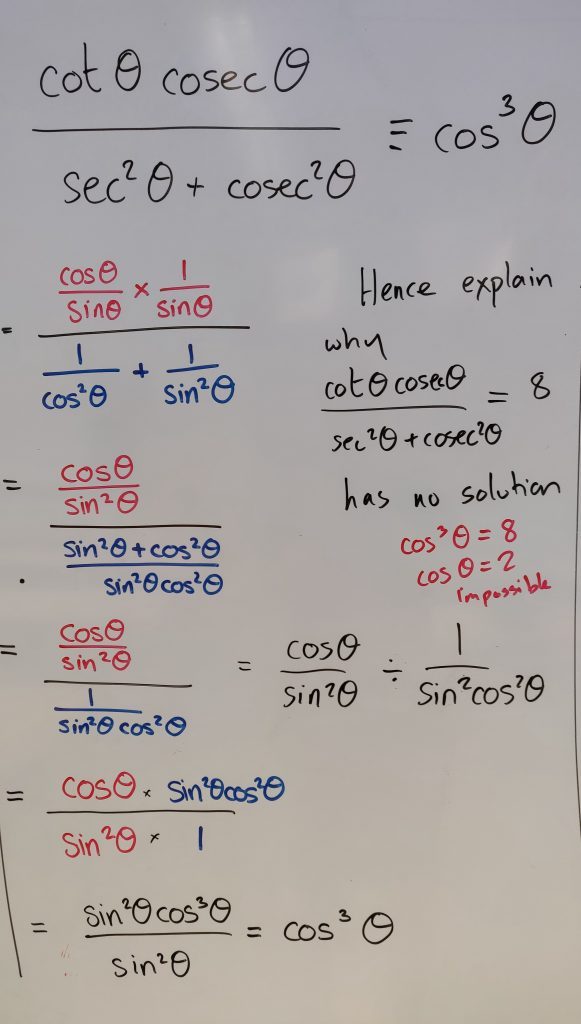Image credit: Mathssandpit

When I discussed this on the board I used separate colours for the expressions in the numerator and denominator. The class could follow the logic so easily. It’s probably my most successful introduction to this topic. I saw that some students used highlighter on their notes after I’d gone through it, so they could track the solution.

The second type of question we looked at was solving a trigonometric equation. The straight forward expansion was all in one colour, but the roots of the quadratic were highlighted in different colours. The reasoning behind this was that students often solve half the quadratic and neglect the other impossible solution. Our exam board likes to see students consider the other solution and formally reject it. It makes the solution complete. By using a colour, the impossible solution stands out and reminds students to provide a whole solution.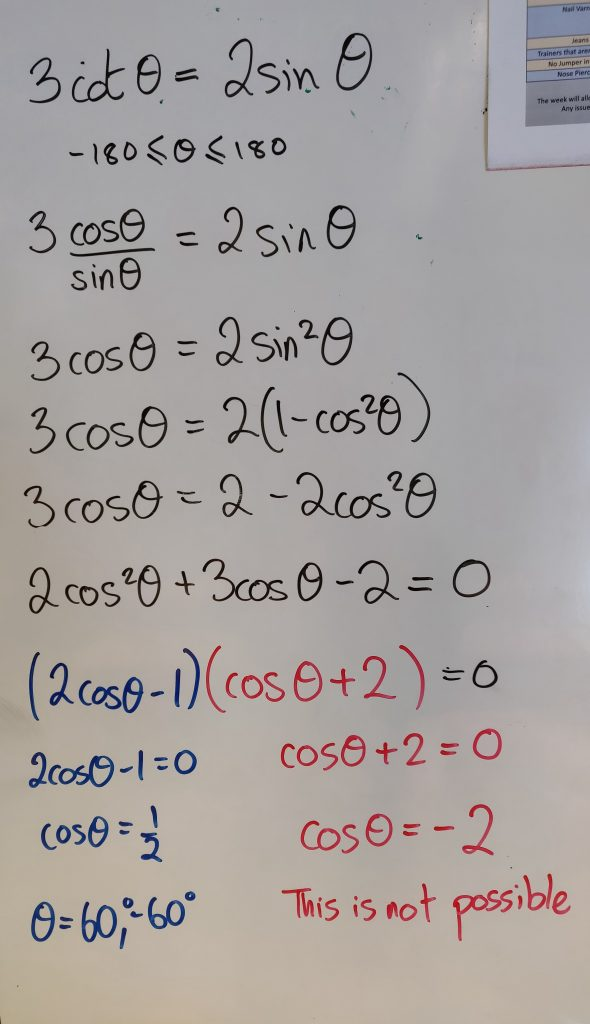Image credit: Mathssandpit

So when you are planning for misconceptions at A-level, remember that coloured pens aren’t just for younger students.

Update: 22nd October

The brilliant Mr B has shared how he uses colour to identify the forces in perpendicular directions in Mechanics.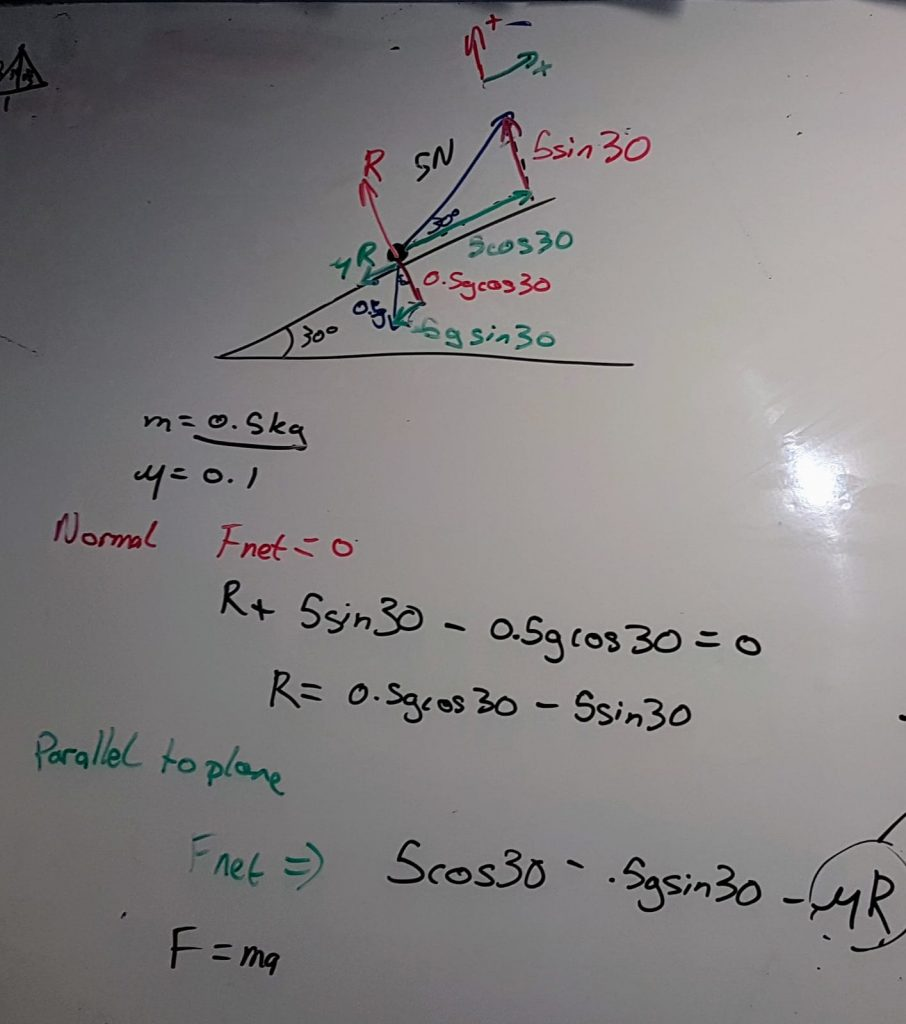# 344. Picture of the day

This student really knows how to make eye-catching notes.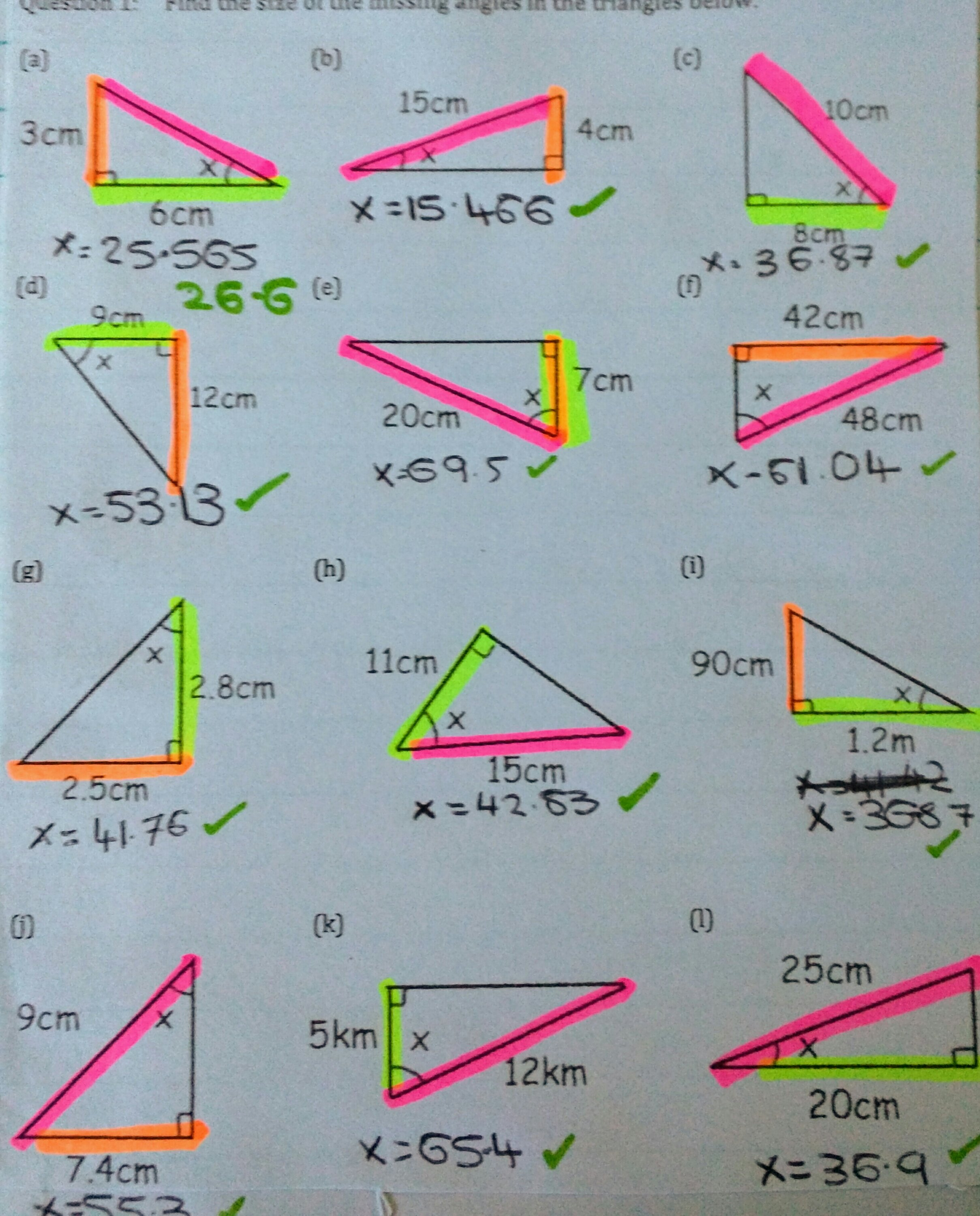There was even a key: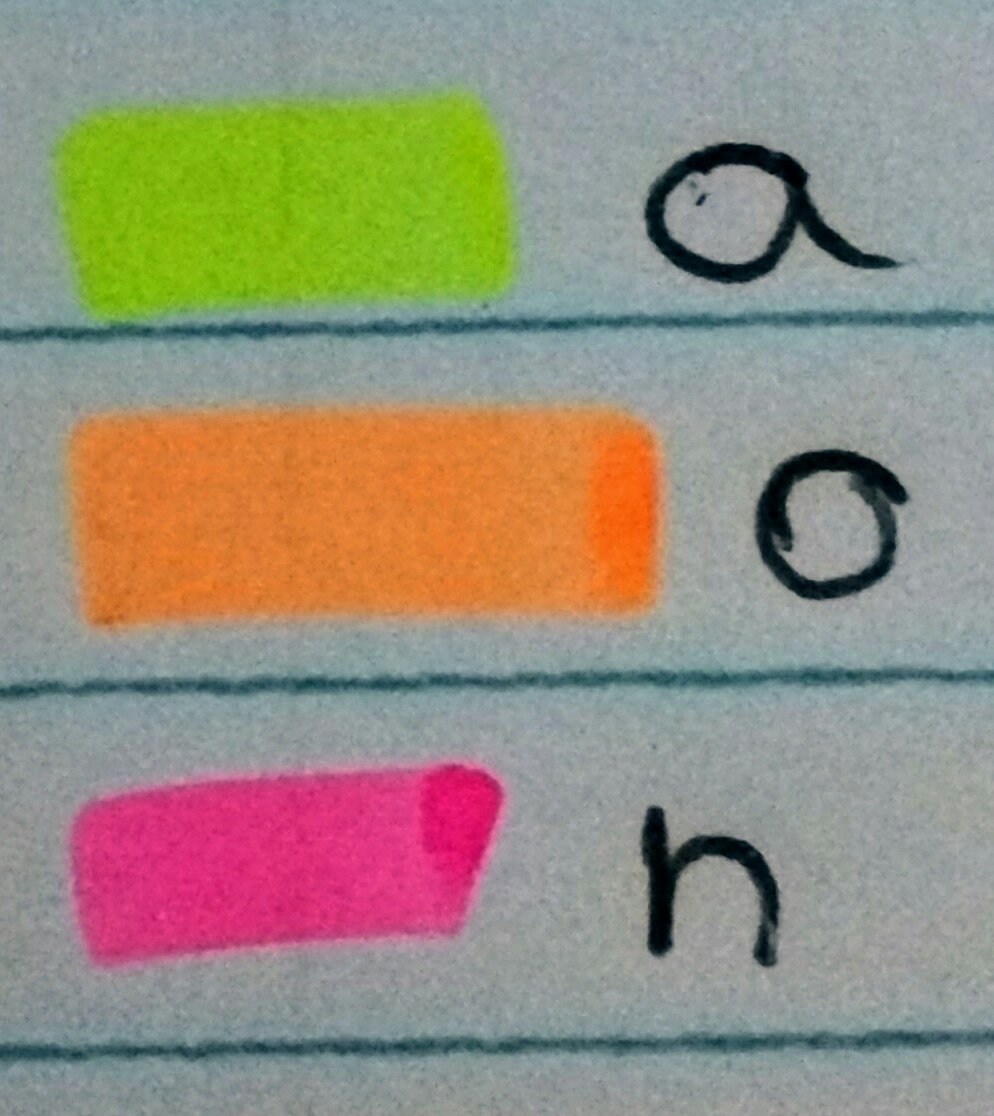# 281. Mathsconf5 resources

Hi to all those who went to Mathsconf5, in Sheffield.

If you liked the proportion snapdragon you can download it here: Proportion Snapdragon

There are instructions for it here: Trigonometry Snapdragon

If you’d like a snapdragon template or instructions on how to fold it click here: http://mathssandpit.co.uk/blog/?p=667

If you want more foldables after the Paper Maths session, run by the lovely @MsSteel_Maths, I can recommend this resource: Foldables by Dinah Zike

# 237. Quick Starter

Don’t you just hate it when students forget basic key skills? Especially those at the higher end of Year 11 or studying A-Level, who should have a better core knowledge. What if there was a magic tool which began to address this issue?

Skills required

• Comparing fractions
• Trigonometric ratios
• Simplifying surds
• Rationalising surds
• Pythagoras

Equipment

You will not need:

• Worksheet
• Powerpoint
• Printer
• Laminator
• Calculator

Magic Tool

• One board, with pen

Activity

Quite simply draw the four diagrams below on the board and ask the following questions: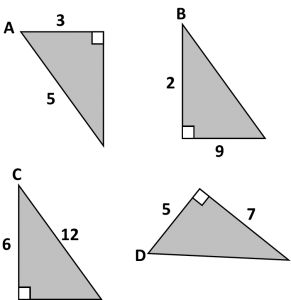1. Which has the largest sine ratio: A or B?
2. Which has the largest cosine ratio: C or D?
3. Which has the smallest tangent ratio: A, B, C or D?
4. Extension: Calculate the missing angles and areas (Calculator allowed)

It takes moments to draw the questions on the board, but the discussion can take some time and addresses several basic skills. You can change the numbers to adjust the level of challenge.

# 215. Another Takeaway

After the warm response the first takeaway homework received, I’ve written another! This time it is about Pythagoras and basic trigonometry – suitable for introductory or revision homework. This one hasn’t yet been trialled, so let me know how it goes.

Pythagoras and Trigonometry homework

# 207. Is it on the formula sheet?

In the Autumn term I put together a booklet of all the Trigonometry and Differentiation rules that you need for the Core 3 (Edexcel) exam. It was a summary of key facts and highlighted what you need to learn vs what is on the formula sheet. The original post was 155.Trigonometry&Differention including links to the booklet.

One term on, at the request of students, I’ve produced the same kind of booklet for Core 4 Integration and Differentiation. Even if you don’t do the Edexcel exams, they are still helpful revision tools.

# 155. Trigonometry & DifferentiationMy Year 13 class have just finished C3 and actually asked a very sensible set of questions:

• Which formulae are given to you?
• Which formulae must you learn?
• Which formulae can you work out from given rules?
• How do you prove simple rules?

In response to this, I’ve written a booklet for the Edexcel C3 paper: Trigonometry and Differentiation: What you are given and what you need to learn (docx) ( PDF Version)